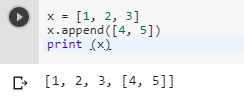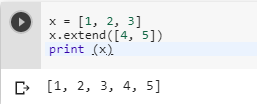3 views
in Python
edited

Can Someone Please tell me the difference between list methods append() and extend() in Python?

+1 vote
by (46k points)
edited by

Append is used to add it’s argument as single element at the end of the list and the length of the list increases by one and it’s time complexity is constant i.e ,0(1) whereas extend increases the length of the list by number of it’s argument, It iterates over it’s argument and adding each element to the list and extending the list.

I am attaching some Syntax of both:

syntax:

# Adds an object (a number, a string or a

# another list) at the end of my_list

my_list.append(object)

Input:

my_list = [AG, 'PD']

my_list.append('AG')

print my_list

Output:

['AG', 'PD', 'AG']

Input

my_list = ['AG', 'for', 1, 2, 3, 4]

my_list.extend('AG')

print my_list

Output:

['AG', 'for', 1, 2, 3, 4, 'A', 'G']

Hope This Helps.

by (10.9k points)
edited

Append(): It adds a new element at the end of the list and increases the length of the list by one.

a= [11, 12, 13]

a.append([14, 15])

print (a)

Output-[11,12,13,[14,15]]

Extend(): It appends elements from iterables at the end of the list and increases the list by the number of elements present in that iterable argument.

a = [11, 12, 13]

a.extend([14, 15])

print (a)

Output-[11,12,13,14,15]

by (1k points)
edited by
Append() adds one element at the end of list.

Extend() takes a single argument and adds it at the end of list
by (106k points)
edited by

The difference between Python's list methods append and extend are as follows which can be understood by the below-mentioned codes:-

append():

The append function is used to append the objects at the end of the list. Below is the piece of code that explains how it works:-

x = [1, 2, 3]

x.append([4, 5])

print (x)extend():

The extend function is used to extend the list by appending elements from the iterable. You can visualize this by looking at the example mentioned below:-

x = [1, 2, 3]

x.extend([4, 5])

print (x)You can use the following video tutorials to clear all your doubts:-

Learn in detail about Python by enrolling in Intellipaat Python Course online and upskill.